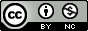Important Note: These materials have been updated for use with version 2.8. As of version 2, pomp syntax has changed substantially. These changes are documented on the pomp website.

## Introduction

This course focuses on the use of models for understanding, predicting, and controlling ecological and epidemiological systems. Models play a central role in this work because they allow us to precisely and quantitatively express our ideas about mechanisms. To the extent our understanding of the important mechanisms is correct, such models are extremely useful in the design of policy. On the other hand, models are useful in uncovering the important mechanisms, inasmuch as we can compare model predictions to data. Specifically, if we can translate competing hypotheses into mathematical models, these can be compared in terms of their ability to correctly capture the patterns seen in data.

In order to fairly compare competing models, we must first try to find out what is the best each model can do. Practically speaking, this means that we have to find the values of the models’ parameters that give the closest correspondence between model predictions and data. Parameter estimation can be challenging even when we are fairly confident in the ability of a single model to explain the dynamics.

### The SIR model

As a simple example for use in this lesson, we’ll focus on the classical SIR model (Kermack and McKendrick 1927). This model’s simplicity allows us to postpone many of the complexities that will arise as we grapple with real data. However, it enjoys the feature that many complexities can be incorporated as modifications and extensions of the model. The model divides a population of hosts into three classes: susceptible, infected, recovered. The model describes how the portion of the population in each of these classes changes with time. Births are modeled as flows from “elsewhere” into the susceptible class; deaths are modeled as flows from the S, I, or R compartment into “elsewhere”. If $$S$$, $$I$$, and $$R$$ refer to the numbers of individuals in each compartment, then these state variables change according to the following system of differential equations: \begin{aligned} \frac{dS}{dt} &= B-\lambda\,S-\mu\,S\\ \frac{dI}{dt} &= \lambda\,S-\gamma\,I-\mu\,I\\ \frac{dR}{dt} &= \gamma\,I-\mu\,R.\\ \end{aligned} Here, $$B$$ is the crude birth rate (births per unit time), $$\mu$$ is the death rate and $$\gamma$$ is the recovery rate. We’ll assume that the force of infection, $$\lambda$$, has the form $\lambda = \beta\,\frac{I}{N},$ so that the risk of infection a susceptible faces is proportional to the prevalence (the fraction of the population that is infected). This is known as the assumption of frequency-dependent transmission.

### Data from an outbreak of measles in Niamey

Biweekly data for outbreaks of measles in three communities within Niamey, Niger (Grais et al. 2006) are provided on the course website. To download and plot the data, do, e.g.,

niamey <- read.csv("http://kingaa.github.io/clim-dis/parest/niamey.csv")
ggplot(niamey,mapping=aes(x=biweek,y=measles,color=community))+
geom_line()+geom_point()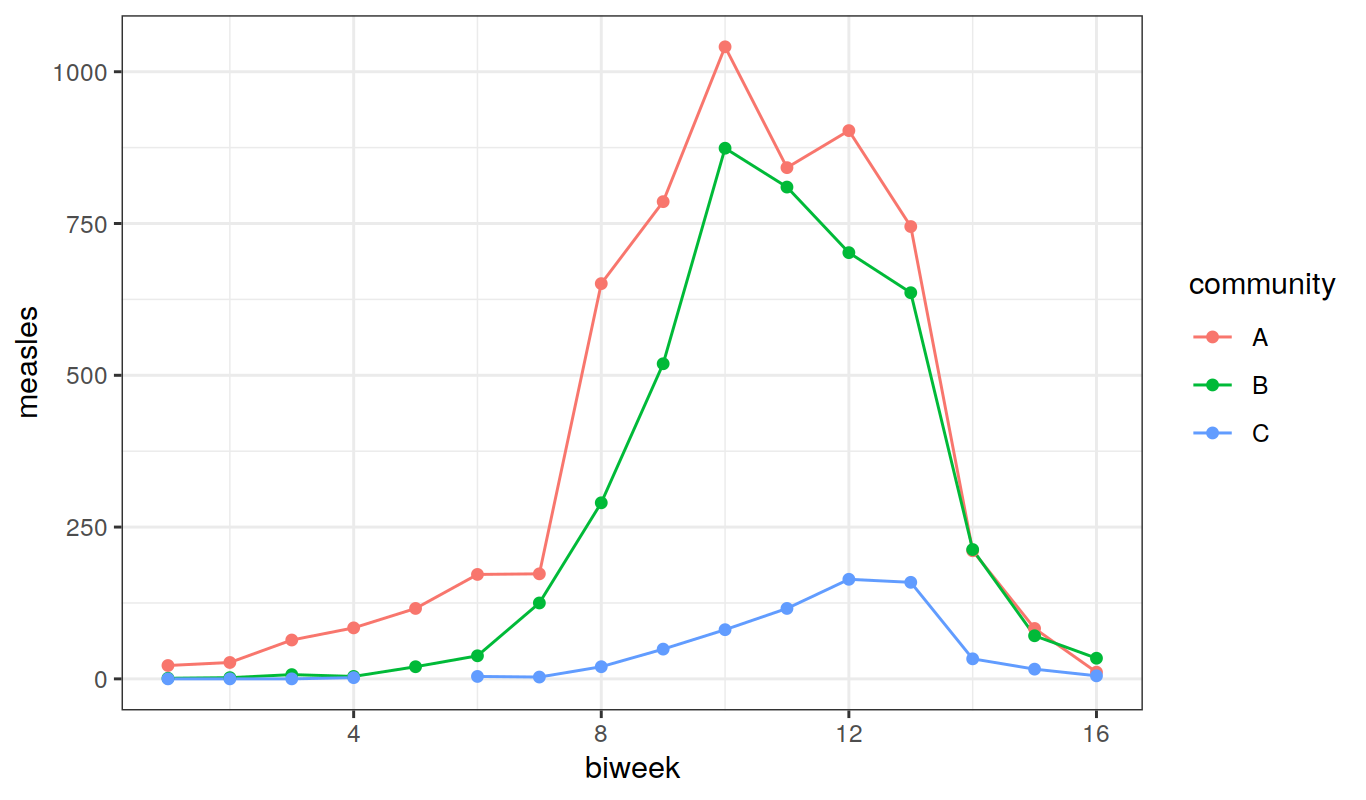### Integrating differential equations with pomp

Although focused on stochastic models, pomp provides facilities for dealing with the important special case of deterministic dynamics. The “Working with ODE models” tutorial shows how deterministic models are implemented and solved.

## Feature-based parameter estimation

A classical approach to the estimation of parameters is to identify informative features of a dataset and then choose parameters in a model so as to match those features. This feature matching approach is sometimes known as the generalized method of moments and is experiencing something of a revival in recent years. Here, we give some examples of this approach in the specific context of a single epidemic curve, modeled as a closed SIR epidemic.

### Estimating $$R_0$$ in an invasion

During the early stages of an SIR outbreak, the number of infected individuals $$I$$ at time $$t$$ is approximately $I(t)\;\approx\;I_0\,e^{(R_0-1)\,(\gamma+\mu)\,t}$ where $$I_0$$ is the (small) number of infectives at time $$0$$, $$\frac{1}{\gamma}$$ is the infectious period, and $$\frac{1}{\mu}$$ is the host lifespan. Taking logs of both sides, we get $\log{I}\;\approx\;\log{I_0}+(R_0-1)\,(\gamma+\mu)\,t,$ which implies that a semi-log plot of $$I$$ vs $$t$$ should be approximately linear with a slope proportional to $$R_0-1$$ and the recovery rate.

Let us plot the Niamey measles data (Grais et al. 2006) from community “A” in this way to see if this is the case.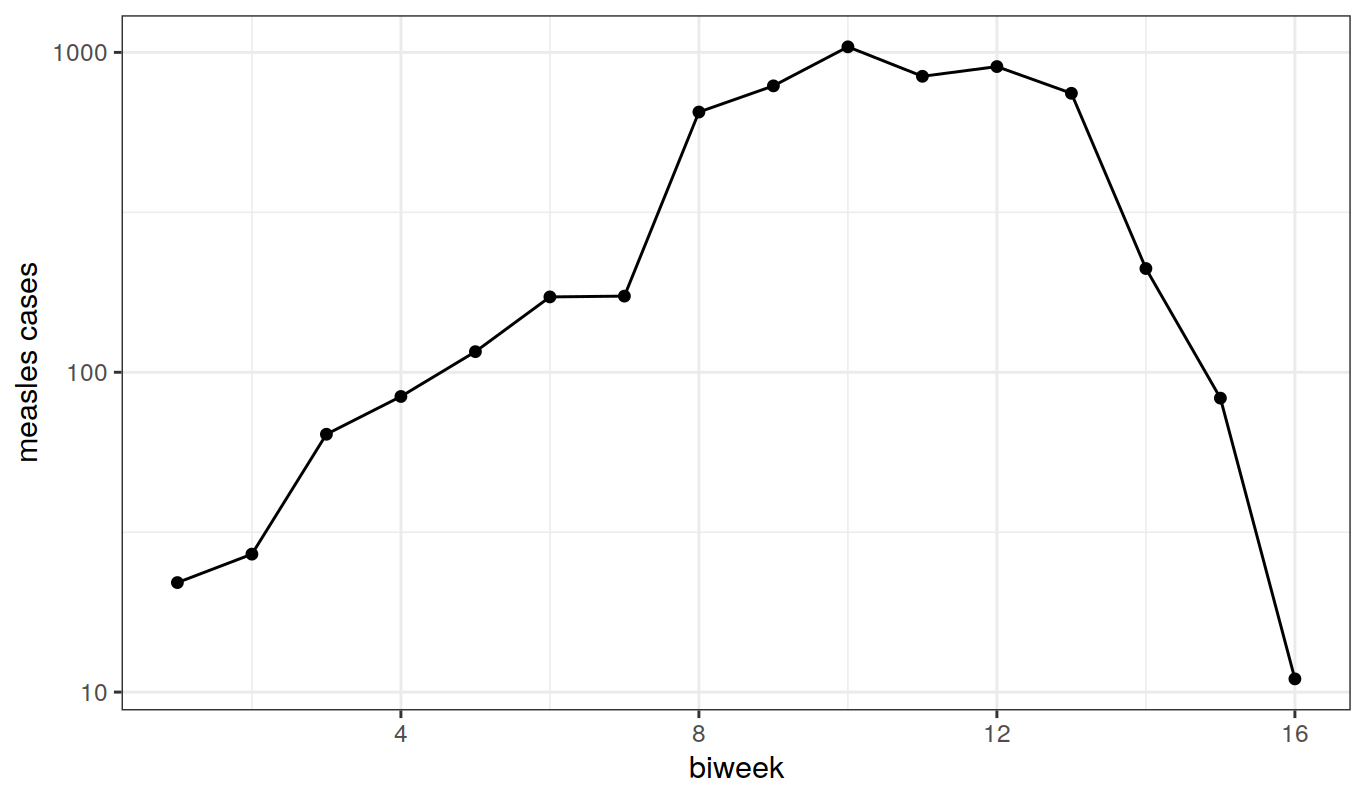Plotted on a log scale, the linearity of the first several data points is evident. This suggests that we can obtain a cheap and cheerful estimate of $$R_0$$ by a simple linear regression:

fit1 <- lm(log(measles)~biweek,data=subset(niamey,biweek<=8&community=="A"))
summary(fit1)
##
## Call:
## lm(formula = log(measles) ~ biweek, data = subset(niamey, biweek <=
##     8 & community == "A"))
##
## Residuals:
##      Min       1Q   Median       3Q      Max
## -0.49040 -0.09510  0.00664  0.12315  0.40282
##
## Coefficients:
##             Estimate Std. Error t value Pr(>|t|)
## (Intercept)  2.61968    0.22727  11.527 2.56e-05 ***
## biweek       0.43200    0.04501   9.599 7.31e-05 ***
## ---
## Signif. codes:  0 '***' 0.001 '**' 0.01 '*' 0.05 '.' 0.1 ' ' 1
##
## Residual standard error: 0.2917 on 6 degrees of freedom
## Multiple R-squared:  0.9389, Adjusted R-squared:  0.9287
## F-statistic: 92.13 on 1 and 6 DF,  p-value: 7.312e-05
coef(fit1)
## (Intercept)      biweek
##   2.6196750   0.4320018
slope <- coef(fit1); slope
##    biweek
## 0.4320018

Now, we know that measles’ infectious period is about 2 wk. Moreover, since this is far shorter than an average human life ($$\mu\ll\gamma$$), we can neglect $$\mu$$ in our estimating equation. Thus our estimate of $$R_0$$ is $\hat{R_{0}}\;=\;\mathrm{slope}/\gamma+1\;\approx\;{0.43}\times{1}+1\;\approx\;{1.4}.$

Our strategy in this case has been to redefine the problem so that it fits a standard form, i.e., we showed how to rearrange the model so that the relevant quantity ($$R_0$$) could be obtained from linear regression. This means that all the usual diagnostics associated with linear regression are also available to check the fit of the model. We can get a rough estimate of the uncertainty in our estimate by looking at the standard errors in our estimator.

coef(summary(fit1))
##              Estimate Std. Error   t value     Pr(>|t|)
## (Intercept) 2.6196750 0.22727156 11.526629 2.562760e-05
## biweek      0.4320018 0.04500648  9.598659 7.312397e-05
slope.se <- coef(summary(fit1))[2,2]
1*slope.se
##  0.04500648

So we reckon we’ve got an error of $$\pm{0.05}$$ in our estimate of $$R_0$$, i.e., we feel pretty confident that $${1.34}<R_{0}<{1.52}$$.

A defect of this method is that it uses only a small amount of the data to compute an important quantity. Moreover, we have to make a subjective judgement as to how much of the data to use. Further, as we use more data, and presumably obtain more precise estimates, we simulataneously get further from the realm where our approximation is valid, which introduces greater bias. Let’s see how our estimates of $$R_0$$ depend on what we choose to be the “initial phase” of the outbreak. Below, we estimate $$R_0$$ and its standard error using the first 2, 3, 4, …, 10 data points. We then plot these estimates with their uncertainties to show this precision-accuracy tradeoff.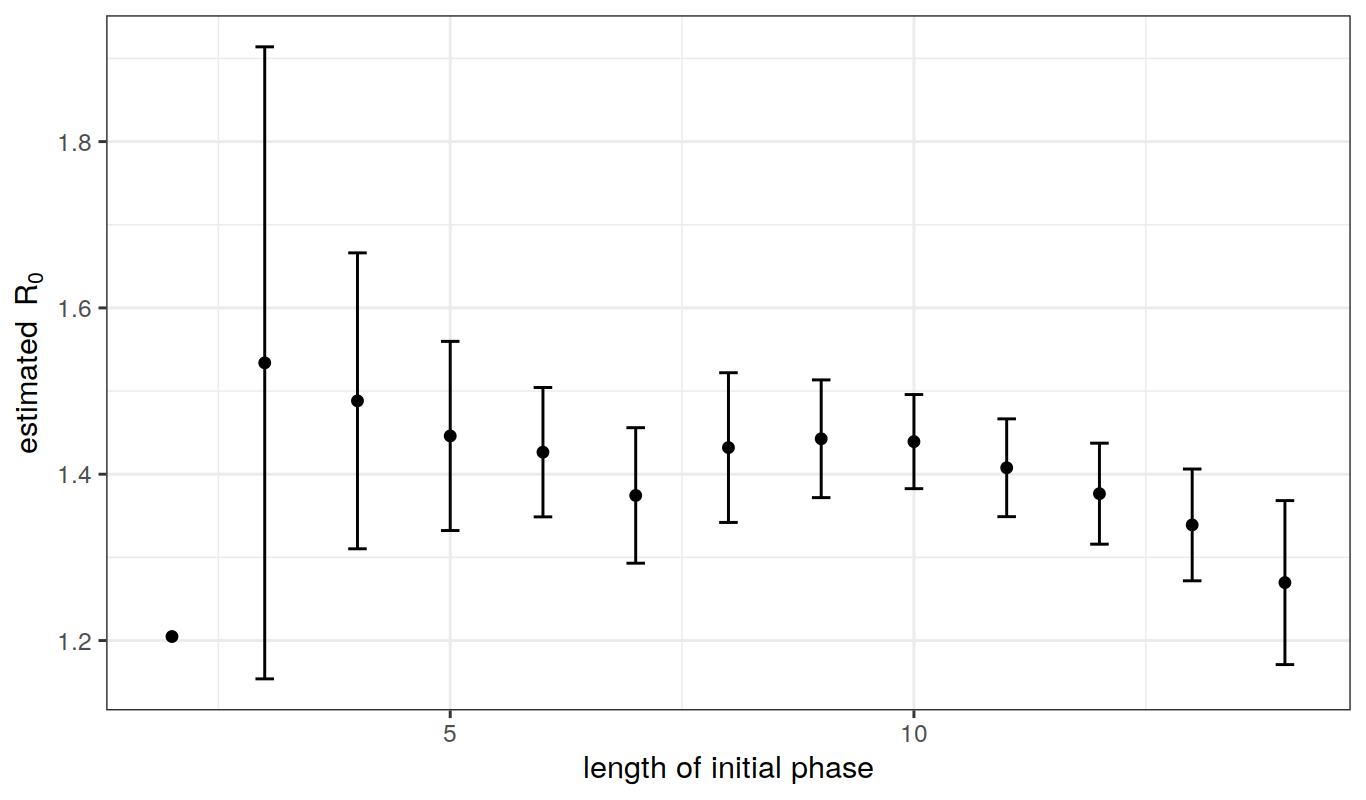### Estimating $$R_0$$ from the final size

If we know the final size of an outbreak and the total population size, we can use the final size equation to estimate $$R_0$$.

##### Exercise: estimating $$R_0$$ for measles in Niamey

Combine the final-size and the invasion rate methods to obtain estimates of $$R_0$$ and $$N$$ using the data from each of the communities of Niamey, assuming that the infectious period is approximately two weeks.

## Fitting deterministic dynamical epidemiological models to data

### Least squares

We now move on to a much more general but considerably more complicated technique for estimating $$R_0$$. The method of least squares gives us a way to quantify the discrepancy between the data and a model’s predictions. We can then search over all possible values of a model’s parameters to find the parameters that minimize this discrepancy.

We’ll illustrate this method using the Niamey data. Since this is a single outbreak, and the number of births and deaths into the population over the course of the outbreak is small relative to the size of the population, we can treat this outbreak as if it were occurring in a closed population.

Thus far, we have only considered deterministic models. In the next lesson, we will begin to think about more realistic models that begin to take into account some aspects of the stochastic nature of real epidemics. For now, under the assumption that the epidemic is deterministic, parameter estimation is a matter of finding the model trajectory that gives the best fit to the data. The first thing we need is a pomp object encoding the model and the data.

pomp(
data=subset(niamey,community=="A",select=-community),
times="biweek",t0=0,
skeleton=vectorfield(
Csnippet("
DS = -Beta*S*I/N;
DI = Beta*S*I/N-gamma*I;
DR = gamma*I;")),
rinit=Csnippet("
S = S_0;
I = I_0;
R = N-S_0-I_0;"),
statenames=c("S","I","R"),
paramnames=c("Beta","gamma","N","S_0","I_0")) -> niameyA

Now we set up a function that will calculate the sum of the squared errors (SSE), or discrepancies between the data and the model predictions.

sse <- function (params) {
x <- trajectory(niameyA,params=params)
discrep <- x["I",,]-obs(niameyA)
sum(discrep^2)
}

To get a sense of what this gives us, let’s vary some parameters and computing the SSE for community “A” of the Niamey data set. To begin with, we’ll assume we know that $$\gamma=1$$ and that the initial numbers of susceptibles, infectives, and recovereds, $$S(0)$$, $$I(0)$$, $$R(0)$$ are known to be 10000, 10, and 20000, respectively. We’ll write a little function that will plug a value of $$\beta$$ into the parameter vector and compute the SSE.

f1 <- function (beta) {
params <- c(Beta=beta,gamma=1,N=50000,S_0=10000,I_0=10)
sse(params)
}
beta <- seq(from=30,to=40,by=0.5)
SSE <- sapply(beta,f1)

We take our estimate, $$\hat{\beta}$$ to be the value of $$\beta$$ that gives the smallest SSE,

beta.hat <- beta[which.min(SSE)]

and plot SSE vs. $$\beta$$:

plot(beta,SSE,type='l')
abline(v=beta.hat,lty=2)What does the SIR model predict at $$\beta=\hat{\beta}$$? We compute the model’s trajectory to find out:

coef(niameyA) <- c(Beta=beta.hat,gamma=1,N=50000,S_0=10000,I_0=10)
x <- trajectory(niameyA,format="data.frame")
ggplot(data=join(as.data.frame(niameyA),x,by='biweek'),
mapping=aes(x=biweek))+
geom_line(aes(y=measles),color='black')+
geom_line(aes(y=I),color='red')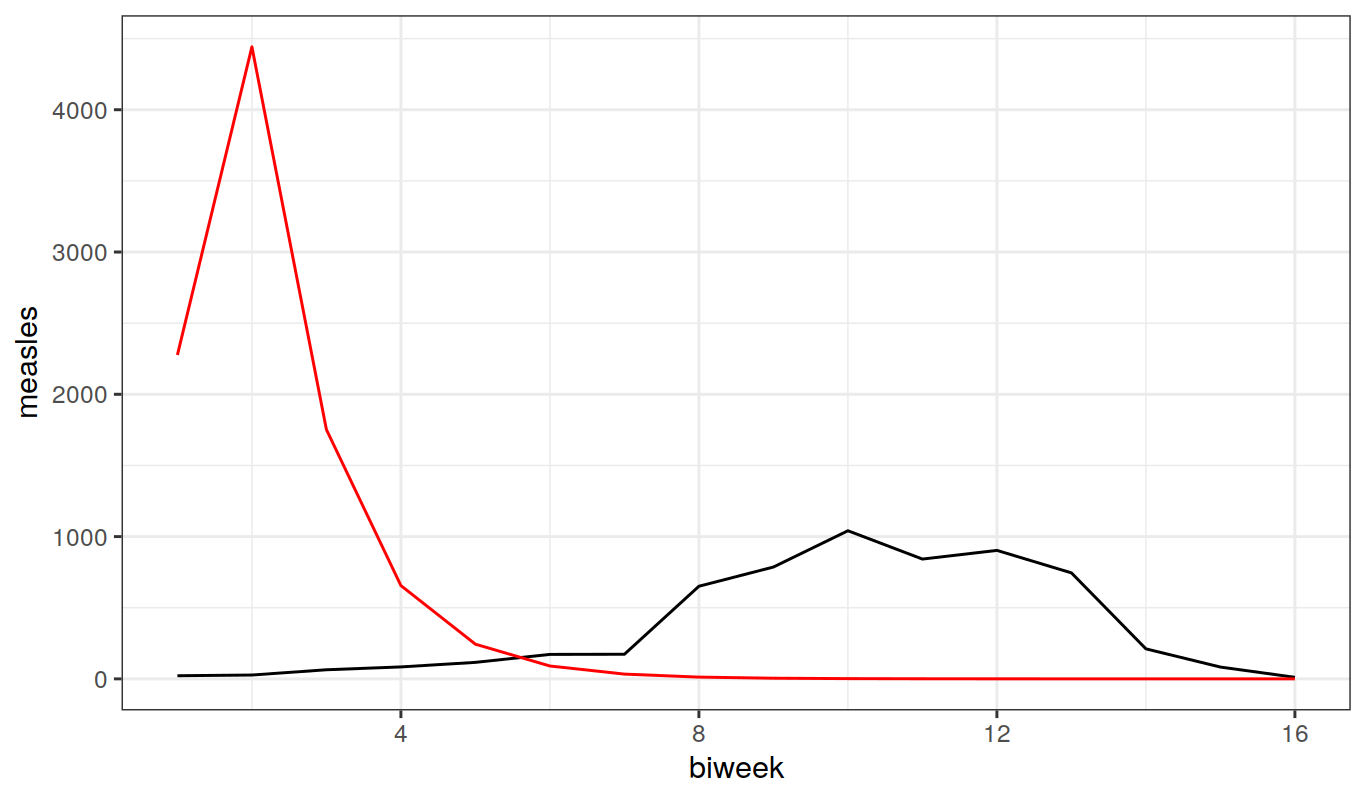beta <- seq(from=0,to=40,by=0.5)
SSE <- sapply(beta,f1)

plot(beta,SSE,type='l')
beta.hat <- beta[which.min(SSE)]
abline(v=beta.hat,lty=2)coef(niameyA,"Beta") <- beta.hat
x <- trajectory(niameyA,format="data.frame")
dat <- join(as.data.frame(niameyA),x,by='biweek')

ggplot(dat,aes(x=biweek))+
geom_line(aes(y=measles),color='black')+
geom_line(aes(y=I),color='red')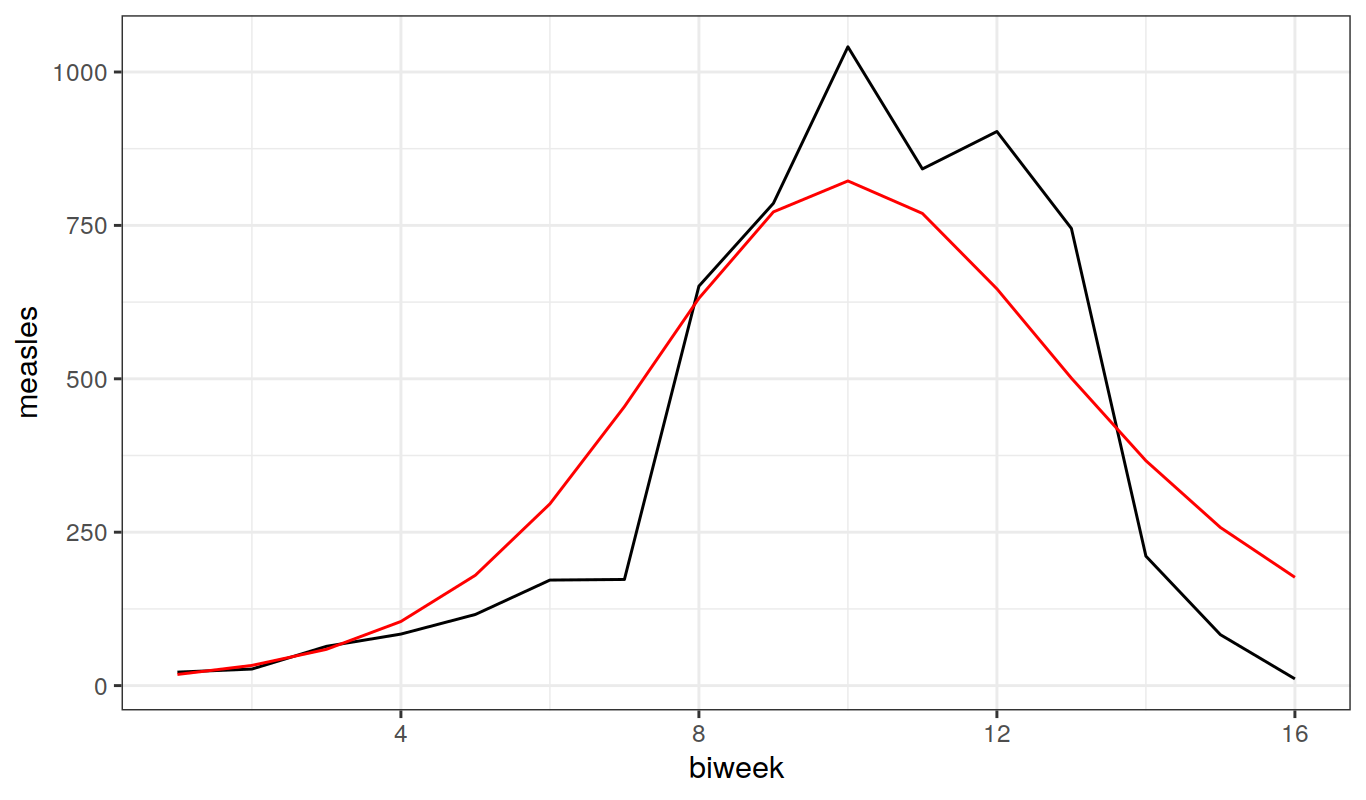##### Exercise: least-squares estimation of $$R_0$$

Use this method to obtain estimates of $$R_0$$ for measles from each of the three communities in Niamey. You may again assume that the infectious period is two weeks.